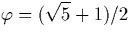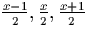Next: Organisation Up: Introduction Previous: Work Performed

# Related Work

The inadequacy of standard digit representations for exact real number computation was first recognised by Brouwer  in 1920. Section 2.2.1 shows why this is the case. In 1937 Alan Turing introduced the notion of a computable function [28,29], and since then, a number of different approaches for exact real arithmetic have been proposed. A brief introduction to some of this related work is presented here. Section 2.2.3 discusses some of the representations used by these authors in more detail.

Avizienis  considers a signed digit representation, as does Wiedmer  when he discusses computation with infinite objects represented as words which may be of infinite length. Wiedmer presents an algorithm for the addition of two signed decimal numbers represented using an infinite signed decimal representation.

Gosper  discusses the use of continued fractions for numerical calculations, and this work is continued by Vuillemin  who uses this representation for exact real arithmetic. Kornerup and Matula  also use continued fractions for real arithmetic, and consider the use of redundant binary strings to representation the coefficients these fractions.

Nielsen and Kornerup  discuss a representation of numbers as potentially infinite strings of signed digits, and define various classes of computable functions on them. They also introduce a floating point style representation not dissimilar to the one used in this project (see section 3.4).

Boehm et al  discuss a number of representations, including the signed digit and continued fraction approaches, and there and in  consider a representation of reals as functions mapping an integer specifying the precision required as n digits in base b to a rational correct to the specified precision. Boehm and Cartwright  implement algorithms using this and other representations, and discuss their relative performance.

Valérie Ménissier-Morain  gives some extremely striking examples of the problems with floating point arithmetic, and summarises approaches to solving these problems. She uses a representation of reals using a sequence of (dyadic) rational numbers which is different from the one used here, and gives algorithms for the usual arithmetic operations and transcendental functions.

Pietro di Gianantonio [8,9] works extensively with digit representations, considering the signed digits used here in addition to a similar one which instead of using three digits in base two, uses two digits whose base is the golden ratio. Algorithms for the usual arithmetic operations in the golden ration notation are given. He also considers an extension of the idealised functional programming language PCF representing reals using the compositions of the linear maps.

Alex Simpson  uses the signed digits and digits formed from dyadic rationals (the two representations used here) to define algorithms for function integration and function minimum and maximum. These algorithms are implemented in this work.

Martín Escardó  uses a representation of reals as an infinite sequence of nested intervals with rational endpoints, and develops an extension to the language PCF which is related to the work of di Gianantonio. He also considers the signed digit representation in  and describes a surprising method by which a pair of numerals may be normalised such that their numerical and lexicographic orderings (which are normally unrelated) can be made to coincide.

Abbas Edalat, Peter Potts, Martín Escardó  consider another extension to the language PCF using Möbius transforms, a type of linear fractional transformation on extended real numbers. Such transformations have the property that their composition can be computed neatly using matrix multiplication. Edalat and Potts [10,24] continue the work of Gosper, Vuillemin, and Nielsen and Kornerup, and develop algorithms for the usual arithmetic operations and transcendental functions

Reinhold Heckmann [14,15] analyses the convergence of the algorithms of Edalat and Potts, and the problems of a swelling representation size.

Interestingly, an on-line calculator for exact real arithmetic demonstrating written by Peter John Potts demonstrating the Linear Fractional Transformation approach available. This calculator available through a Java interface on the World Wide Web at http://theory.doc.ic.ac.uk/ pjp/RealClient.html, but appears to connect to a C++ server.

Philipp Sünderhauf  discusses the benefits of incremental and non-incremental representations, and the creation of hybrid representations to achieve the benefits of each.

Although several of these authors discuss the signed digit representation, none state or prove any operations other than addition using the signed digit stream representation adopted here. This, and the goal of implementing Alex Simpson's functional operations, motivates the work performed here.Next: Organisation Up: Introduction Previous: Work Performed
Martin Escardo
5/11/2000